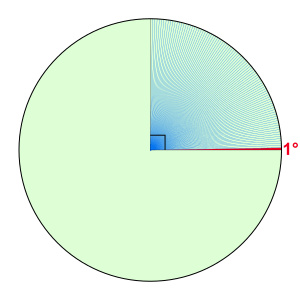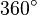# Degree (angle) facts for kids

Kids Encyclopedia Facts
Quick facts for kids
DegreeOne degree (shown in red) and 89 degrees (shown in blue)
General information
Unit system Non-SI accepted unit
Unit of Angle
Symbol ° or deg
Conversions
1 ° in ... ... is equal to ...
turns    1360 turn
gons    109g

In mathematics, a degree is a common way to measure plane angle. It is written with the symbol$^{\circ}$, where$360^{\circ}$ corresponds to the entire circle.

It is not an SI unit. The SI uses radian to measure plane angle. However, according to the SI brochure, it is an accepted unit by SI.

## History

The actual reason of choosing the degree as a way to measure plane angle is unknown. One theory says that it is related with the fact that a year is approximately 360 days. Some ancient calendars, for example the Persian calendar and the Babylonian calendar, used 360 days for a year.

Another theory says that the Babylonians divided the circle using the angle of an equilateral triangle. The angle was then divided into 60 parts. This is because they used sexagesimal or base-60 numeral system.

## Related pagesDegree (angle) Facts for Kids. Kiddle Encyclopedia.